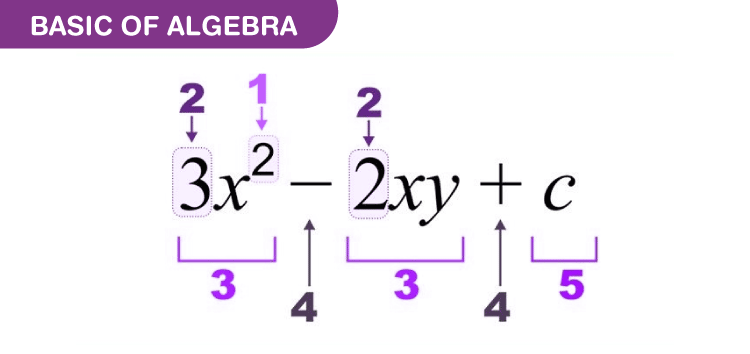# Basic Of Algebra

Basics of Algebra cover the simple operation of mathematics like addition, subtraction, multiplication, and division involving both constant as well as variables. For example, x+10 = 0. This introduces an important algebraic concept known as equations. The algebraic equation can be thought of as a scale where the weights are balanced through numbers or constants.

Algebra is the branch of Maths which uses alphabetical letters to find unknown numbers. These letters are also called variables. The values which are known in the given expression such as numbers are called constants. Though in higher classes, students will learn the concept of algebra at the potential level. But when we speak about its basics, it covers the general algebraic expressions, formulas and identities, which are used to solve many mathematical problems. Let us learn here the basic concept of algebra with the help of some terminology, formulas, rules, examples and solved problems.In the above-given equation, the letters x and y are the unknown variables which we have to determine. Whereas 3 and 2 are the numerical values. c denotes the constant term.

## Basic Algebra

The algebra for class 6 covers all the basic concepts. Terms related to basic algebra skills are mentioned below.

1. Exponent
2. Expression
3. Polynomial (Monomial, binomial and trinomial)
4. Like terms and Unlike terms
5. Constants

An equation is a statement which implies two same identities separated by “=” sign. Whereas an expression is a group of different terms separated by ‘+’ or ‘-‘ sign.

Like terms are those terms whose variables and their exponents are same.

### Basic Algebra Rules

The basic algebra rules are mentioned below:

• The Symmetry rule
• The commutative rules
• Two rules for equation

### Basic Algebra Operations

The general arithmetic operations performed in the case of algebra are:

• Subtraction: x – y
• Multiplication: xy
• Division: x/y or x ÷ y

where x and y are the variables.

The order of these operations will follow the BODMAS rule, which means the terms inside the brackets are considered first. Then, roots and exponents are operated on second priority. Solve all the division and multiplication operations and later addition and subtraction.

### Basic Algebra Formula

The general formulas used in algebra to solve algebraic equations and find the values of unknown variables are given here:

• a2 – b2 = (a – b)(a + b)
• (a+b)2 = a2 + 2ab + b2
• a2 + b2 = (a – b)2 + 2ab
• (a – b)2 = a2 – 2ab + b2
• (a + b + c)2 = a2 + b2 + c2 + 2ab + 2ac + 2bc
• (a – b – c)2 = a2 + b2 + c2 – 2ab – 2ac + 2bc
• (a + b)3 = a3 + 3a2b + 3ab2 + b3
• (a – b)3 = a3 – 3a2b + 3ab2 – b3

These fomulas are used in higher secondary classes. Students can find algebra formulas for class 8, along with class 9, class 10, class 11 and class 12 here.

Some of the basic algebra worksheets are given below containing basic math algebra questions.

### Basic Algebra Examples

Q 1: Find y, when, y + 15 = 30

Solution: y = 30 – 15

y = 15

Q 2 : Find x, when, 9x = 63

Ans. x = 63/9

x = 7

Q.3: If x/7 = 21, then find x.

Solution: Given x/7 = 21

or x = 21 x 7

x = 147

### Practice Problems

• Solve x+12 = 6
• Find the value of z, if 23z + 3 = 10
• Solve 2y – 8 = 5y